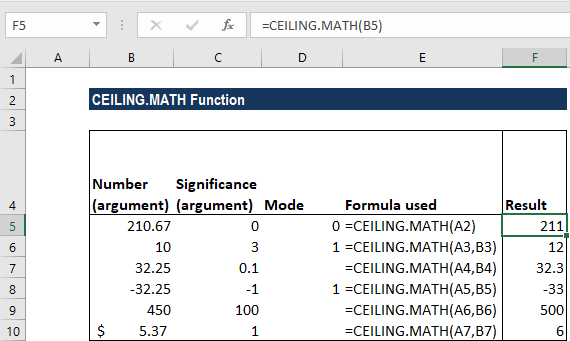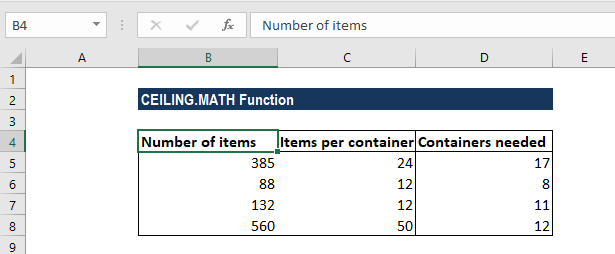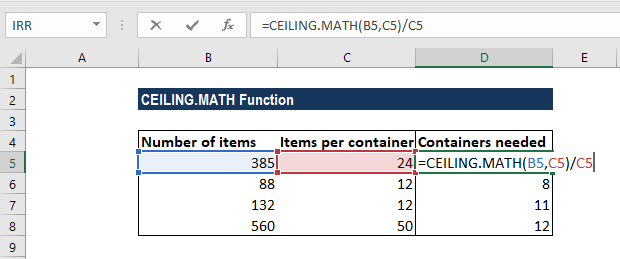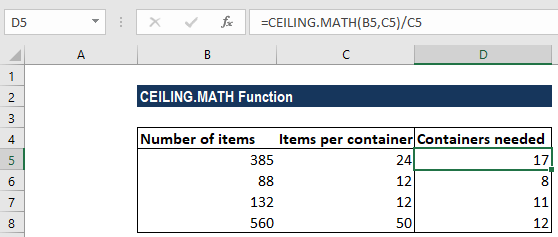# CEILING.MATH Function

Returns a number that is rounded up to the nearest integer or multiple of significance

## What is the CEILING.MATH Function?

The CEILING.MATH Function is categorized under Excel Math and Trigonometry functions. it will return a number that is rounded up to the nearest integer or multiple of significance. The function was introduced in MS Excel 2013.

As a financial analyst, we can use the CEILING.MATH function in setting the pricing after currency conversion, discounts, etc. When preparing financial models, it helps us round up the numbers as per the requirement.

### Formula

=CEILING.MATH(number, [significance], [mode])

The CEILING.MATH function uses the following arguments:

1. Number (required argument) – This is the value that we wish to round off.
2. Significance (optional argument) – This specifies the multiple of significance to round the supplied number to.

If we omit the argument, it takes the default value of 1. That is, it will round up to the nearest integer. Significance will ignore the arithmetic sign. Remember that by default, the significance argument is +1 for positive numbers and -1 for negative numbers.

1. Mode (optional argument) – This will reverse the direction of rounding for negative numbers only.
• If the mode argument is equal to zero, negative numbers are rounded up towards zero.
• If the mode argument is equal to any other numeric value, negative numbers are rounded up away from zero.

### How to use the CEILING.MATH Function in Excel?

To understand the uses of the CEILING.MATH function, let’s consider a few examples:

#### Example 1

Let’s see the results from the function when we provide the following data:

Number (argument)Significance (argument)ModeResultRemarks
210.67211As the [significance] argument is omitted, it takes on the default value of 1.
103112The function rounds up to nearest multiple of 3. Even though mode is 1 but as the number is positive, the mode argument will not affect the result.
32.250.132.3It rounded up away from zero.
-32.25-11-33It rounds -32.25 down (away from 0) to the nearest integer that is a multiple of 1 with a mode of 1, which reverses rounding direction away from zero.
450100500It rounded up to the nearest multiple of 100.
\$5.3716It rounded up to the nearest multiple of 6.

The formula used and results in MS Excel are shown in the screenshot below:#### Example 2

Suppose we wish to know how many containers we will need to hold a given number of items. The data given to us is shown below:The items per container indicate the number of items that can be held in a container.

The formula we will use is =CEILING.MATH(A2,B2). It rounds up A2 to the nearest multiple of B2 (that is items per container). The value derived will then be divided by the number of containers. For example, in the second row, =CEILING.MATH(385,24)/24, 385 will be rounded to a multiple of 24 and the result will be divided by 24.We get the result below:### A few notes about the CEILING.MATH Function

1. #VALUE error – Occurs when any of the arguments is non-numeric.
2. Instead of the CEILING.MATH function, we can use the FLOOR.MATH function to round down to the nearest integer or significant figure. We can also use the MROUND function to round to a desired multiple or the ROUND function to round to a specified number of digits.
3. CEILING.MATH is actually a combination of the CEILING function and the CEILING.PRECISE function.

Thanks for reading CFI’s guide to important Excel functions! By taking the time to learn and master these functions, you’ll significantly speed up your financial analysis. To learn more, check out these additional CFI resources:

• Excel Functions for Finance